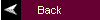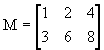MATLAB Tutorial1. MATLAB Basics

MATLAB is started by clicking the mouse on the appropriate icon and is ended by typing exit or by using the menu option. After each MATLAB command, the "return" or "enter" key must be depressed.

A. Definition of Variables

Variables are assigned numerical values by typing the expression directly, for example, typing

a = 1+2

yields: a = 3

The answer will not be displayed when a semicolon is put at the end of an expression, for example type a = 1+2;.

MATLAB utilizes the following arithmetic operators:

 + addition - subtraction * multiplication / division ^ power operator ' transpose

A variable can be assigned using a formula that utilizes these operators and either numbers or previously defined variables. For example, since a was defined previously, the following expression is valid

b = 2*a;

To determine the value of a previously defined quantity, type the quantity by itself:

b

yields: b = 6

If your expression does not fit on one line, use an ellipsis (three or more periods at the end of the line) and continue on the next line.

c = 1+2+3+...
5+6+7;

There are several predefined variables which can be used at any time, in the same manner as user-defined variables:

 i sqrt(-1) j sqrt(-1) pi 3.1416...

For example,

y= 2*(1+4*j)

yields: y = 2.0000 + 8.0000i

There are also a number of predefined functions that can be used when defining a variable. Some common functions that are used in this text are:

 abs magnitude of a number (absolute value for real numbers) angle angle of a complex number, in radians cos cosine function, assumes argument is in radians sin sine function, assumes argument is in radians exp exponential function

For example, with y defined as above,

c = abs(y)

yields: c = 8.2462

c = angle(y)

yields: c = 1.3258

With a=3 as defined previously,

c = cos(a)

yields: c = -0.9900

c = exp(a)

yields: c = 20.0855

Note that exp can be used on complex numbers. For example, with y = 2+8i as defined above,

c = exp(y)

yields: c = -1.0751 + 7.3104i

which can be verified by using Euler's formula:

c = exp(2)cos(8) + je(exp)2sin(8)

back to list of contents

B. Definition of Matrices

MATLAB is based on matrix and vector algebra; even scalars are treated as 1x1 matrices. Therefore, vector and matrix operations are as simple as common calculator operations.

Vectors can be defined in two ways. The first method is used for arbitrary elements:

v = [1 3 5 7];

creates a 1x4 vector with elements 1, 3, 5 and 7. Note that commas could have been used in place of spaces to separate the elements. Additional elements can be added to the vector:

v(5) = 8;

yields the vector v = [1 3 5 7 8]. Previously defined vectors can be used to define a new vector. For example, with v defined above

a = [9 10];
b = [v a];

creates the vector b = [1 3 5 7 8 9 10].

The second method is used for creating vectors with equally spaced elements:

t = 0:.1:10;

creates a 1x101 vector with the elements 0, .1, .2, .3,...,10. Note that the middle number defines the increment. If only two numbers are given, then the increment is set to a default of 1:

k = 0:10;

creates a 1x11 vector with the elements 0, 1, 2, ..., 10.

Matrices are defined by entering the elements row by row:

M = [1 2 4; 3 6 8];

creates the matrixThere are a number of special matrices that can be defined:

 null matrix: M = [ ]; nxm matrix of zeros: M = zeros(n,m); nxm matrix of ones: M = ones(n,m); nxn identity matrix: M = eye(n);

A particular element of a matrix can be assigned:

M(1,2) = 5;

places the number 5 in the first row, second column.

In this text, matrices are used only in Chapter 12; however, vectors are used throughout the text. Operations and functions that were defined for scalars in the previous section can also be used on vectors and matrices. For example,

a = [1 2 3];
b = [4 5 6];
c = a + b

yields:
c =  5  7
9

Functions are applied element by element. For example,

t = 0:10;
x = cos(2*t);

creates a vector x with elements equal to cos(2t) for t = 0, 1, 2, ..., 10.

Operations that need to be performed element-by-element can be accomplished by preceding the operation by a ".". For example, to obtain a vector x that contains the elements of x(t) = tcos(t) at specific points in time, you cannot simply multiply the vector t with the vector cos(t). Instead you multiply their elements together:

C. General Information

Matlab is case sensitive so "a" and "A" are two different names.

Comment statements are preceded by a "%".

On-line help for MATLAB can be reached by typing help for the full menu or typing help followed by a particular function name or M-file name. For example, help cos gives help on the cosine function.

The number of digits displayed is not related to the accuracy. To change the format of the display, type format short e for scientific notation with 5 decimal places, format long e for scientific notation with 15 significant decimal places and format bank for placing two significant digits to the right of the decimal.

The commands who and whos give the names of the variables that have been defined in the workspace.

The command length(x) returns the length of a vector x and size(x) returns the dimension of the matrix x.

back to list of contents

D. M-files

M-files are macros of MATLAB commands that are stored as ordinary text files with the extension "m", that is filename.m. An M-file can be either a function with input and output variables or a list of commands. All of the MATLAB examples in this textbook are contained in M-files that are available at the MathWorks ftp site, ftp.mathworks.com in the directory pub/books/heck.

The following describes the use of M-files on a PC version of MATLAB. MATLAB requires that the M-file must be stored either in the working directory or in a directory that is specified in the MATLAB path list. For example, consider using MATLAB on a PC with a user-defined M-file stored in a directory called "\MATLAB\MFILES". Then to access that M-file, either change the working directory by typing cd\matlab\mfiles from within the MATLAB command window or by adding the directory to the path. Permanent addition to the path is accomplished by editing the \MATLAB\matlabrc.m file, while temporary modification to the path is accomplished by typing path(path,'\matlab\mfiles') from within MATLAB.

The M-files associated with this textbook should be downloaded from the MathWorks ftp site and copied to a subdirectory named "\MATLAB\KAMEN" and then this directory should be added to the path. The M-files that come with MATLAB are already in appropriate directories and can be used from any working directory.

As example of an M-file that defines a function, create a file in your working directory named yplusx.m that contains the following commands:

function z = yplusx(y,x)
z = y + x;

The following commands typed from within MATLAB demonstrate how this M-file is used:

x = 2;
y = 3;
z = yplusx(y,x)

MATLAB M-files are most efficient when written in a way that utilizes matrix or vector operations. Loops and if statements are available, but should be used sparingly since they are computationally inefficient. An example of the use of the command for is

for k=1:10,
x(k) = cos(k);
end

This creates a 1x10 vector x containing the cosine of the positive integers from 1 to 10. This operation is performed more efficiently with the commands

k = 1:10;
x = cos(k);

which utilizes a function of a vector instead of a for loop. An if statement can be used to define conditional statements. An example is

if(a <= 2),
b = 1;
elseif(a >=4)
b = 2;
else
b = 3;
end

The allowable comparisons between expressions are >=, <=, <, >, ==, and ~=.

Several of the M-files written for this textbook employ a user-defined variable which is defined with the command input. For example, suppose that you want to run an M-file with different values of a variable T. The following command line within the M-file defines the value:

T = input('Input the value of T: ')

Whatever comment is between the quotation marks is displayed to the screen when the M-file is running, and the user must enter an appropriate value.

This tutorial is available as a supplement to the textbook Fundamentals of Signals and Systems Using Matlab by Edward Kamen and Bonnie Heck, published by Prentice Hall. The tutorial is designed for students using either the professional version of MATLAB (ver. 5.0) with the Control Systems Toolbox (ver. 4.0) and the Signal Processing Toolbox (ver. 4.0), or using the Student Edition of MATLAB (ver. 5.0). For more information on MATLAB, contact The Mathworks, Inc.